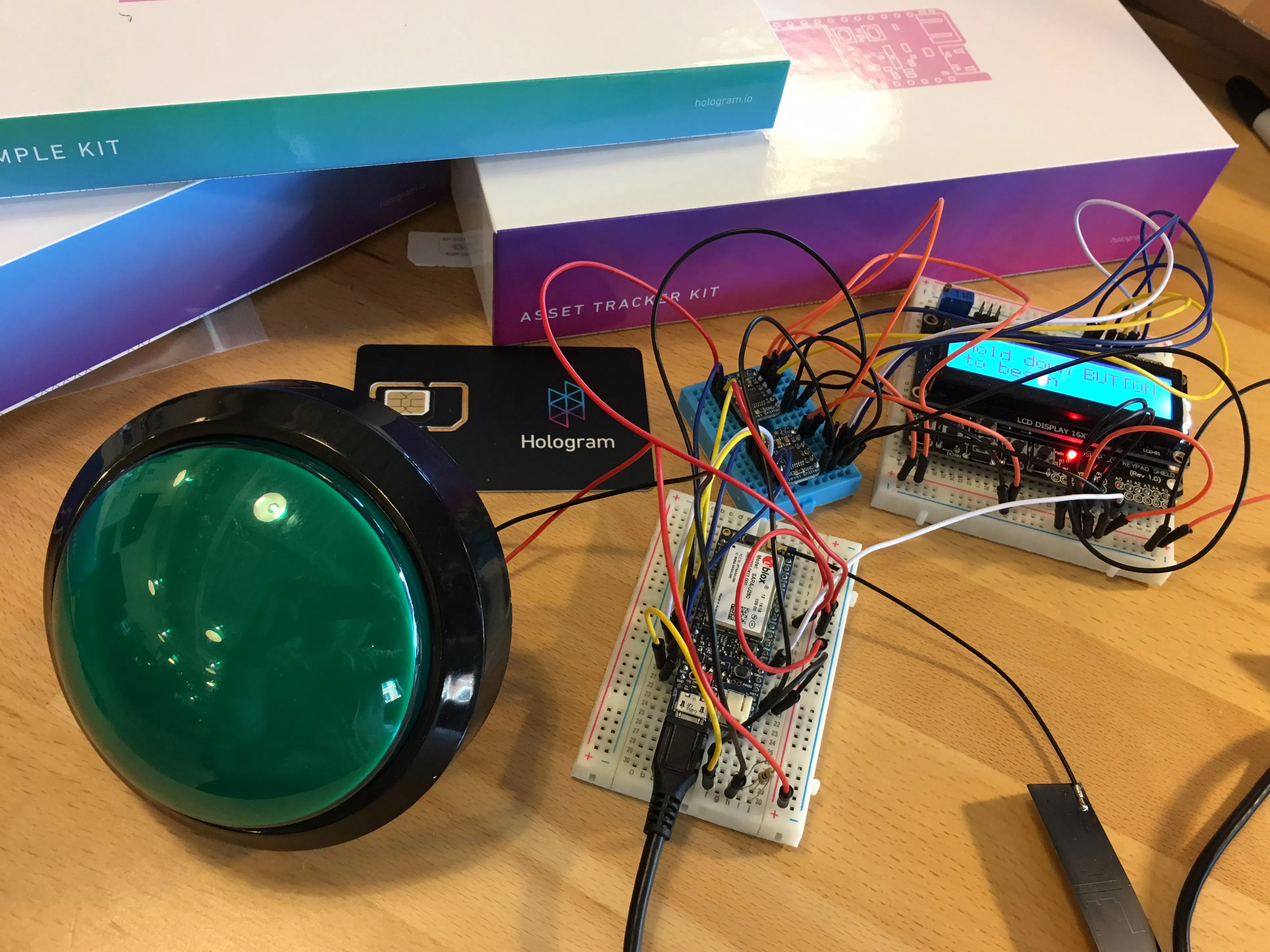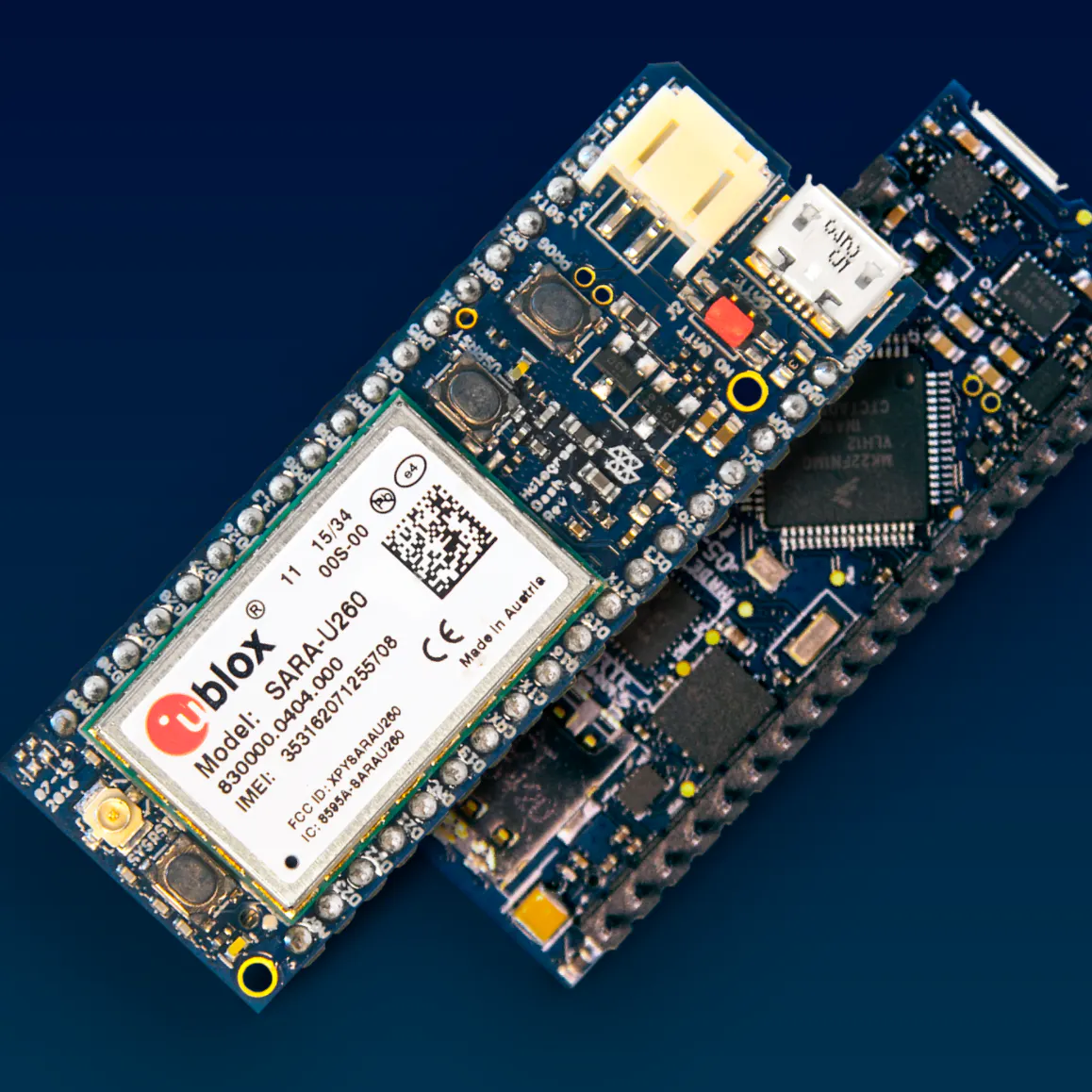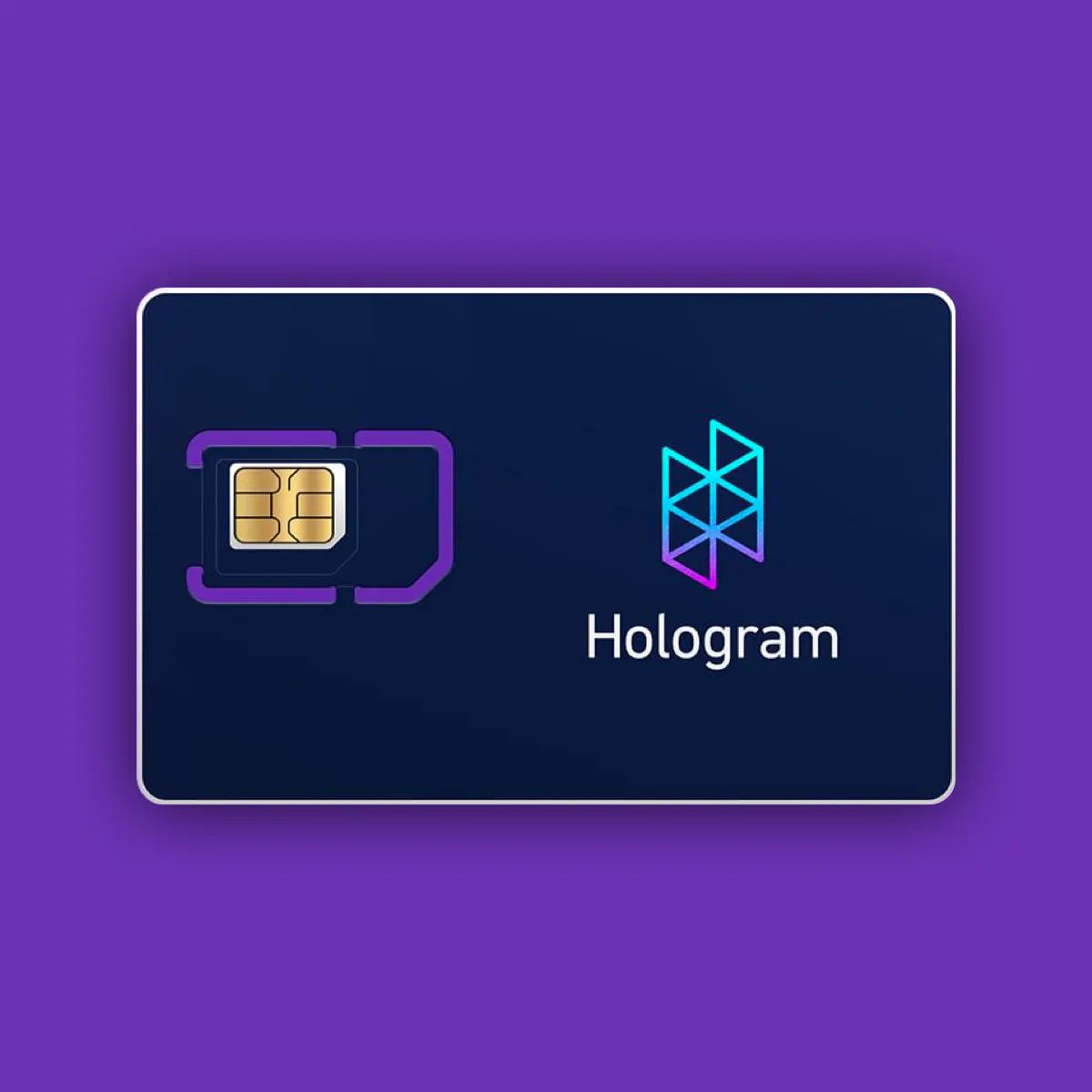This is a cellular-connected internet button placed in a remote location. Hit the button, enter your initials, and get recognized.

BeginnerFull instructions provided1 hour2,202## Things used in this project

### Hardware componentsHologram Dash The Hologram Dash v1.1 is the brains and connectivity for this project
×1Hologram Global IoT SIM Card M2M / IoT SIM that connects this device directly to the Hologram cloud
×1
×1
×1Arduino UNO Only used for a 5V output
×1
 LCD Display - 16x2 Made by Osepp - works at 5V
×1Resistor 1k ohm
×1Jumper wires (generic)
×25

### Software apps and online servicesHologram Data RouterArduino IDE

## Code

### Dash Sketch on Arduino IDE

Arduino
This is the main sketch for the Aggro-Crag-in-Real-Life-Button
```//Sample using LiquidCrystal library
#include <LiquidCrystal.h>

// select the pins used on the LCD panel
//LiquidCrystal lcd(8, 9, 4, 5, 6, 7);  //original library pins
LiquidCrystal lcd(D17, D18, D11, D12, D13, D14);

//set up number of sends to cloud
int numSends = 0; // count number of sends

//set up the big green button
int big_button_in = 0;

//network connection boot sequence variables
int bootSequence = 0;
char currChar;
String tempBuffer;
int plusSigns = 0;

// define some values used by the panel and buttons
int lcd_key     = 0;
#define btnRIGHT  0
#define btnUP     1
#define btnDOWN   2
#define btnLEFT   3
#define btnSELECT 4
#define btnNONE   5

{
// my buttons when read are centered at these valies: 0, 144, 329, 504, 741
// we add approx 50 to those values and check to see if we are close
if (adc_key_in > 1000) return btnNONE; // We make this the 1st option for speed reasons since it will be the most likely result
if (adc_key_in < 60)   return btnRIGHT;
if (adc_key_in < 200)  return btnUP;
if (adc_key_in < 450)  return btnDOWN;
if (adc_key_in < 640)  return btnLEFT;
if (adc_key_in < 970)  return btnSELECT;
return btnNONE;  // when all others fail, return this...
}

//define alphabet array
char alphaArray = {'A', 'B', 'C', 'D', 'E', 'F', 'G', 'H', 'I', 'J', 'K', 'L', 'M', 'N', 'O', 'P', 'Q', 'R', 'S', 'T', 'U', 'V', 'W', 'X', 'Y', 'Z'};
int currentAlphaPosition = 0;

int currentCursorPosition = 0;
char initials = {'A','A','A'};

int cursorTracker = {0,0,0};
int sentToCloudFlag = 0;

int nextAlphaPosition(int current, int lcd_key){
int next = current;

if(lcd_key == btnUP){
next = current + 1;
}
else if(lcd_key == btnDOWN){
next = current - 1;
}

if (next == 26){
next = 0;
}

if (next == -1){
next = 25;
}

return next;

}

int nextCursorPosition(int current, int lcd_key){
int next = current;

if(lcd_key == btnRIGHT){
next = current + 1;
}
else if(lcd_key == btnLEFT){
next = current - 1;
}

if (next == 3){
next = 0;
}

if (next == -1){
next = 2;
}

return next;

}

//debounce setup
// Variables will change:
int buttonState;             // the current reading from the input button
int lastButtonState = btnNONE;   // the previous reading from the input pin

// the following variables are long's because the time, measured in miliseconds,
// will quickly become a bigger number than can be stored in an int.
long lastDebounceTime = 0;  // the last time the output pin was toggled
long debounceDelay = 50;    // the debounce time; increase if the output flickers

//lcd state machine flags
int scene1 = 0;
int scene2 = 0;
int scene3 = 0;
int scene4 = 0;
int scene5 = 0;

void setup()
{
lcd.begin(16, 2);              // start the library
lcd.setCursor(0,0);
lcd.print("Booting..."); // print a simple message

Serial.begin(9600);
SerialCloud.begin(115200);
}

void loop()
{

//lcd.setCursor(9,1);            // move cursor to second line "1" and 9 spaces over
//lcd.print(millis()/1000);      // display seconds elapsed since power-up

//connection boot
if(bootSequence == 0){
char currChar;

while (SerialCloud.available()) {

if (currChar == '+') {
plusSigns++;
} else {
plusSigns = 0;
}

if (plusSigns == 3) {
scene1=1;
bootSequence=1;
lcd.clear();
}

SerialUSB.write(currChar);
Serial2.write(currChar);
}
}

if (scene1 == 1){
delay(1000);
Serial.println("Entering Scene 1");
lcd.setCursor(0,0);
lcd.print("Hold down BUTTON");

lcd.setCursor(0,1);
lcd.print("to begin");

if (big_button_in >  500){
scene1 = 0;
scene2 = 1;
lcd.clear();
}
}

if (scene2 == 1){
lcd.setCursor(0,0);
lcd.print("Congrats! Press");

lcd.setCursor(0,1);
lcd.print("SELECT");

//just recopy a bunch of code because refactoring is hard...

// If the switch changed, due to noise or pressing:
if (lcd_key != lastButtonState) {
// reset the debouncing timer
lastDebounceTime = millis();
}

if ((millis() - lastDebounceTime) > debounceDelay) {

// if the button state has changed:
if (lcd_key != buttonState) {
buttonState = lcd_key;
if (lcd_key == btnSELECT){
scene2 = 0;
scene3 = 1;
lcd.clear();
lcd.setCursor(0,0);
Serial.println("Select pressed");
}
}
}

// it'll be the lastButtonState:
lastButtonState = lcd_key;
}

if (scene3 == 1){
lcd.setCursor(0,1);            // move to the begining of the second line

lcd.setCursor(0,0);
lcd.print("Enter initials");

// If the switch changed, due to noise or pressing:
if (lcd_key != lastButtonState) {
// reset the debouncing timer
lastDebounceTime = millis();
}

if ((millis() - lastDebounceTime) > debounceDelay) {

// if the button state has changed:
if (lcd_key != buttonState) {

//Serial.println("button state has changed");
buttonState = lcd_key;

currentCursorPosition = nextCursorPosition(currentCursorPosition, lcd_key);
currentAlphaPosition = nextAlphaPosition(cursorTracker[currentCursorPosition], lcd_key);
cursorTracker[currentCursorPosition] = currentAlphaPosition;

initials[currentCursorPosition] = alphaArray[currentAlphaPosition];
Serial.print(initials);
Serial.print(initials);
Serial.println(initials);

if (lcd_key == btnRIGHT){
Serial.print("Right");
Serial.println(currentCursorPosition);
}
else if (lcd_key == btnUP){
Serial.print("Up");
Serial.println(alphaArray[currentAlphaPosition]);
}
else if (lcd_key == btnDOWN){
Serial.print("Down");
Serial.println(alphaArray[currentAlphaPosition]);
}
else if (lcd_key == btnLEFT){
Serial.print("Left");
Serial.println(currentCursorPosition);
}
else if (lcd_key == btnSELECT){
Serial.print("Select");
scene3 = 0;
scene4 = 1;
}
}

}

// it'll be the lastButtonState:
lastButtonState = lcd_key;

lcd.setCursor(0,1);
lcd.print(initials);
lcd.setCursor(1,1);
lcd.print(initials);
lcd.setCursor(2,1);
lcd.println(initials);
}

if (scene4 == 1){
lcd.setCursor(0,0);
lcd.print("Sending. Go to");

lcd.setCursor(0,1);
lcd.print("hologram.io");
String cloudInitials = initials;

// Try to send data 3 times to cloud
if(numSends < 3) {
SerialUSB.println("Sending a message to the Cloud...");
SerialCloud.println(cloudInitials);
SerialUSB.println(cloudInitials);
SerialUSB.println("Message sent!");
numSends++; // increase the number-of-sends counter
}

if(numSends >= 3){
scene4 = 0;
scene5 = 1;
}

// it'll be the lastButtonState:
lastButtonState = lcd_key;
}

if (scene5 == 1){
lcd.clear();
lcd.setCursor(0,0);
lcd.print("Sending. Go to");

lcd.setCursor(0,1);
lcd.print("hologram.io");

delay(7500);

lcd.clear();
lcd.setCursor(0,0);
lcd.print("Resetting...");

lcd.setCursor(0,1);
lcd.print("Goodbye!");
delay(5000);
lcd.clear();
scene5 = 0;
scene1 = 1;

//Reset variables
currentAlphaPosition = 0;

currentCursorPosition = 0;
initials='A';
initials='A';
initials='A';

cursorTracker=0;
cursorTracker=0;
cursorTracker=0;
sentToCloudFlag = 0;
numSends = 0;

}

}
```

## Credits

### Derrick Wolbert

1 project • 1 follower

### Sheena Martin

1 project • 0 followers
Content Coordinator for Hologram.io

### benstr 🚀

18 projects • 98 followers
Connecting the world with Soracom.io and AWS.